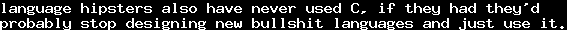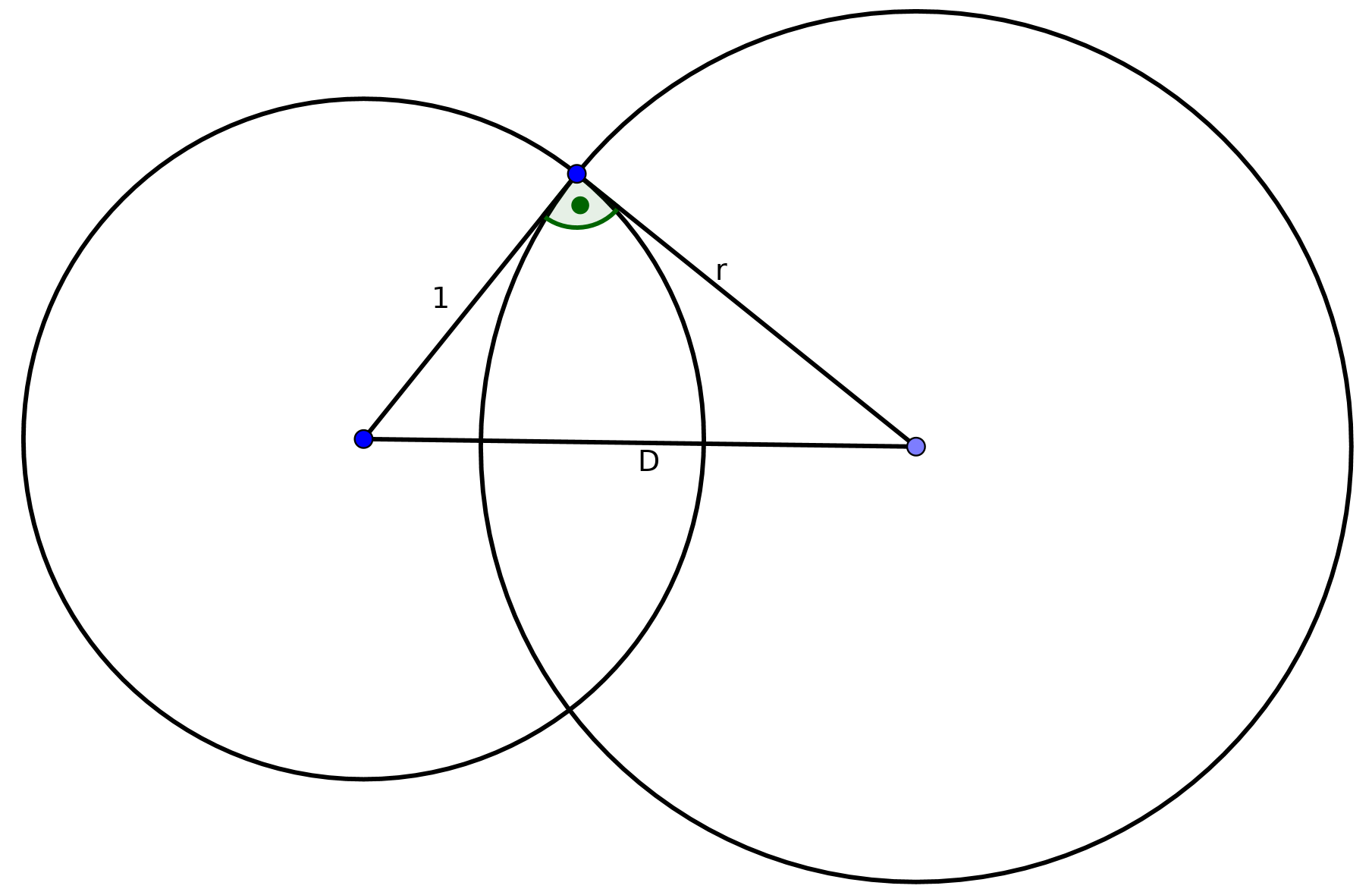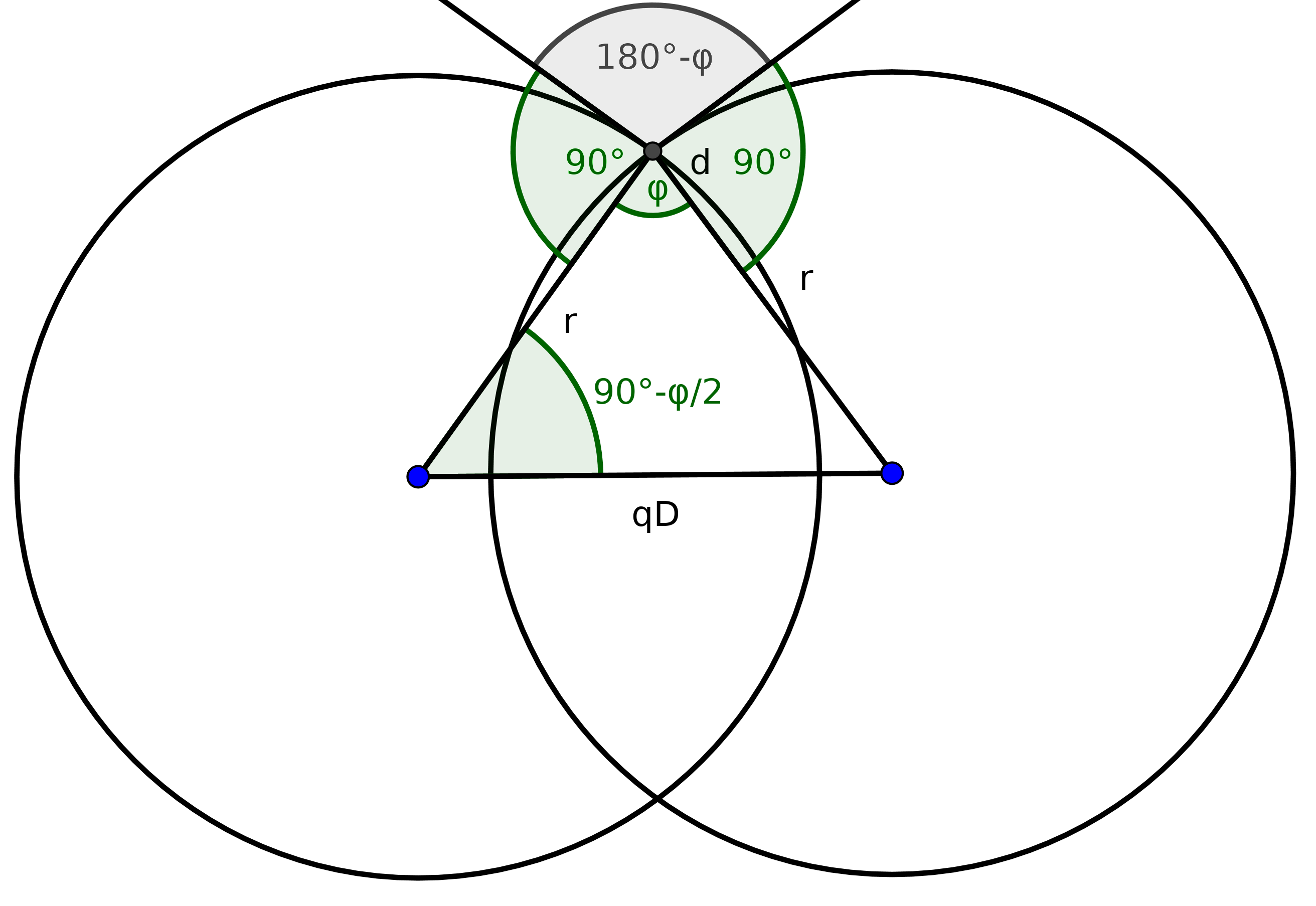# Hyperbolic PolygonsMon, 19 Feb 2018 12:59:38 GMT

I was searching for a tutorial for how to generate hyperbolic tessellations programmatically. Since I found none, I guess it is a worthwile thing to use some of my free time to write about my own approaches. This is not a scientific text. Probably there are better solutions to this, and I would be interested in them, so feel free to comment.

I do not yet have a satisfactory way to give unique coordinates to every tile as for example HyperRogue does. If you know how to do this, feel free to send a comment. It has to do with Coxeter Groups.

We will work on the Poincaré Disk Model. In the poincare disk model, the hyperbolic space is the unit circle, and lines are circles and lines perpendicular to this unit circle.For circles perpendicular to the unit circle, it is easy to see that we have, whereis the distance between the two centers, because they form a right-angled triangle. Hence, this holds for every line in hyperbolic space that is a circle in euclidean space.

If we want to generate a regular tessellation of regular-gons, such that at every vertexsuch-gons meet, we need to generate an-gon with inner angle. We know that the centers of the hyperbolic lines must form an-gon in euclidean space. The side length of the regular-gon in euclidean space is.We now consider two such circles. Their center distance should be. Their radiiare equal. Fix one of their two intersection points and draw the radii through these intersection points. Together with the segment between their two centers, this forms an isosceles triangle. The anglebetween the two radii is complementary to the angle of the normals through this point, and therefore we know that. The other two angles in this isosceles triangle, let's call them, satisfy.

Now we know by basic trigonometry that the height of the triangle is given by, and the bottom sidesatisfies.

Inserting the above relation betweenand, we getwhereTo generate the sides of the polygon, draw circles with radiusaround the centersfor.

To find the vertices of the hyperbolic-gon, just calculate the intersection points between neighbouring circles, and choose the one with distanceto the origin. See this thread about how to calculate intersections.